# Archimedes’ cattle problem

Archimedes’ cattle problem (or the problema bovinum or problema Archimedis) is a problem in Diophantine analysis, the study of polynomial equations withinteger solutions. Attributed to Archimedes, the problem involves computing the number of cattle in a herd of the sun god from a given set of restrictions. The problem was discovered by Gotthold Ephraim Lessing in a Greek manuscript containing a poem of forty-four lines, in the Herzog August Library in WolfenbüttelGermany in 1773.

## History

In 1769, Gotthold Ephraim Lessing was appointed librarian of the Herzog August Library in WolfenbüttelGermany, which contained many Greek and Latin manuscripts. A few years later, Lessing published translations of some of the manuscripts with commentaries. Among them was a Greek poem of forty-four lines, containing an arithmetical problem which asks the reader to find the number of cattle in the herd of the god of the sun. The name of Archimedes appears in the title of the poem, it being said that he sent it in a letter to Eratosthenes to be investigated by the mathematicians of Alexandria. The claim that Archimedes authored the poem is disputed, though, as no mention of the problem has been found in the writings of the Greek mathematicians.

## Problem

The problem, from an abridgement of the German translations published by Georg Nesselmann in 1842, and by Krumbiegel in 1880, states:

Compute, O friend, the number of the cattle of the sun which once grazed upon the plains of Sicily, divided according to color into four herds, one milk-white, one black, one dappled and one yellow. The number of bulls is greater than the number of cows, and the relations between them are as follows:

White bullsblack bulls + yellow bulls,
Black bulls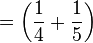dappled bulls + yellow bulls,
Dappled bullswhite bulls + yellow bulls,
White cowsblack herd,
Black cowsdappled herd,
Dappled cowsyellow herd,
Yellow cowswhite herd.

If thou canst give, O friend, the number of each kind of bulls and cows, thou art no novice in numbers, yet can not be regarded as of high skill. Consider, however, the following additional relations between the bulls of the sun:

White bulls + black bulls = a square number,
Dappled bulls + yellow bulls = a triangular number.

If thou hast computed these also, O friend, and found the total number of cattle, then exult as a conqueror, for thou hast proved thyself most skilled in numbers.

## Solution

The first part of the problem can be solved readily by setting up a system of equations. If the number of white, black, dappled, and yellow bulls are written asand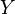, and the number of white, black, dappled, and yellow cows are written as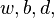and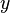, the problem is simply to find a solution to: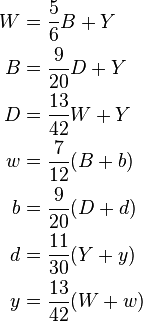which is a system of seven equations with eight unknowns. It is indeterminate, and has infinitely many solutions. The least positive integers satisfying the seven equations are:which is a total of 50,389,082 cattle and the other solutions are integral multiples of these. Note that the first four numbers are multiples of 4657, a value which will appear repeatedly below.

The general solution to the second part of the problem was first found by A. Amthor in 1880. The following version of it was described by H. W. Lenstra, based on Pell’s equation: the solution given above for the first part of the problem should be multiplied bywhere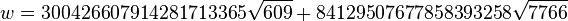and j is any positive integer. Equivalently, squaring w results in,where {u,v} are the fundamental solutions of the Pell equation,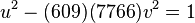The size of the smallest herd that could satisfy both the first and second parts of the problem is then given by j = 1, and is about(first solved by Amthor). Modern computers can easily print out all digits of the answer. This was first done at the University of Waterloo, in 1965 by Hugh C. Williams, R. A. German, and Charles Robert Zarnke. They used a combination of the IBM 7040 and IBM 1620 computers.

## Pell Equation

The constraints of the second part of the problem are straightforward and the actual Pell equation that needs to be solved can easily be given. First, it asks that B+Wshould be a square, or using the values given above,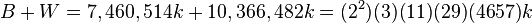thus one should set k = (3)(11)(29)(4657)q2 for some integer q. That solves the first condition. For the second, it requires that D+Y should be a triangular number,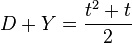Solving for t,Substituting the value of D+Y and k and finding a value of q2 such that the discriminant of this quadratic is a perfect square p2 entails solving the Pell equation,Amthor’s approach discussed in the previous section was essentially to find the smallest v such that it is integrally divisible by 2*4657. The fundamental solution of this equation has more than 100,000 digits.

Source: Wikipedia